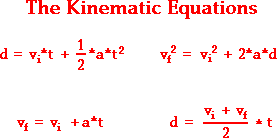1-D Kinematics - Lesson 6 - Describing Motion with Equations

# The Kinematic EquationsThe goal of this first unit of The Physics Classroom has been to investigate the variety of means by which the motion of objects can be described. The variety of representations that we have investigated includes verbal representations, pictorial representations, numerical representations, and graphical representations (position-time graphs and velocity-time graphs). In Lesson 6, we will investigate the use of equations to describe and represent the motion of objects. These equations are known as kinematic equations.

There are a variety of quantities associated with the motion of objects - displacement (and distance), velocity (and speed), acceleration, and time. Knowledge of each of these quantities provides descriptive information about an object's motion. For example, if a car is known to move with a constant velocity of 22.0 m/s, North for 12.0 seconds for a northward displacement of 264 meters, then the motion of the car is fully described. And if a second car is known to accelerate from a rest position with an eastward acceleration of 3.0 m/s2 for a time of 8.0 seconds, providing a final velocity of 24 m/s, East and an eastward displacement of 96 meters, then the motion of this car is fully described. These two statements provide a complete description of the motion of an object. However, such completeness is not always known. It is often the case that only a few parameters of an object's motion are known, while the rest are unknown. For example as you approach the stoplight, you might know that your car has a velocity of 22 m/s, East and is capable of a skidding acceleration of 8.0 m/s2, West. However you do not know the displacement that your car would experience if you were to slam on your brakes and skid to a stop; and you do not know the time required to skid to a stop. In such an instance as this, the unknown parameters can be determined using physics principles and mathematical equations (the kinematic equations).

### The BIG 4

The kinematic equations are a set of four equations that can be utilized to predict unknown information about an object's motion if other information is known. The equations can be utilized for any motion that can be described as being either a constant velocity motion (an acceleration of 0 m/s/s) or a constant acceleration motion. They can never be used over any time period during which the acceleration is changing. Each of the kinematic equations include four variables. If the values of three of the four variables are known, then the value of the fourth variable can be calculated. In this manner, the kinematic equations provide a useful means of predicting information about an object's motion if other information is known. For example, if the acceleration value and the initial and final velocity values of a skidding car is known, then the displacement of the car and the time can be predicted using the kinematic equations. Lesson 6 of this unit will focus upon the use of the kinematic equations to predict the numerical values of unknown quantities for an object's motion.

The four kinematic equations that describe an object's motion are:There are a variety of symbols used in the above equations. Each symbol has its own specific meaning. The symbol d stands for the displacement of the object. The symbol t stands for the time for which the object moved. The symbol a stands for the acceleration of the object. And the symbol v stands for the velocity of the object; a subscript of i after the v (as in vi) indicates that the velocity value is the initial velocity value and a subscript of f (as in vf) indicates that the velocity value is the final velocity value.

Each of these four equations appropriately describes the mathematical relationship between the parameters of an object's motion. As such, they can be used to predict unknown information about an object's motion if other information is known. In the next part of Lesson 6 we will investigate the process of doing this.

Next Section:

Follow Us